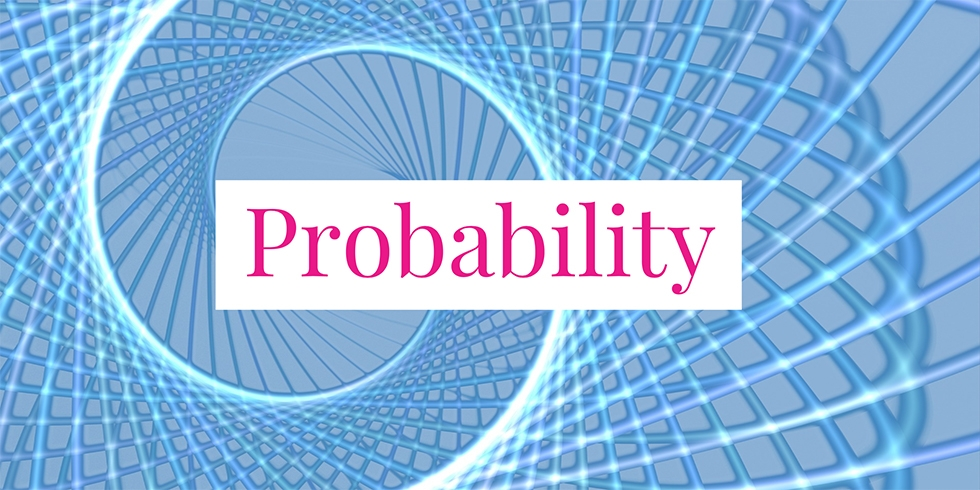# Successful Probability of a Communication Network Diagram## Problem 742

Consider the network diagram in the figure. The diagram consists of five links and each of them fails to communicate with probability $p$. Answer the following questions about this network.

(1) Determine the probability that there exists at least one path from A to B where every link on the path functions without errors. Express the answer in term of $p$.

(2) Assume that exactly one link has failed. In this case, what is the probability that there is a successful path from A to B, that is, each link on the path has not fail.Add to solve later

## Solution.

### Solution of (1)

If there is path along which no link has failed, then Link 5 must be successful and at least one of the upper subpath (Link 1 and Link 3) or the lower subpath (Link 2 and Link 4) should not fail.
Thus the desired probability is
\begin{align*}
& P(\text{there is a successful path})\\
&= P(\text{the upper or lower subpath is successful}) * P(\text{Link 5 is successful}).
\end{align*}

Furthermore, we have using the property of probability that
\begin{align*}
&P(\text{the upper or lower subpath is successful}) \\
&= 1 – P(\text{both upper and lower subpaths failed})\\
&= 1 – P(\text{upper subpaths failed}) * P(\text{lower subpaths failed})
\end{align*}

Now, the upper subpaths fail if Link 1 or Link 3 fails. That is,
\begin{align*}
&P(\text{upper subpaths failed}) \\
&= 1 – P(\text{Link 1 is successful}) * P(\text{Link 3 is successful})\\
&= 1 – (1 – p) ^2\\
\end{align*}
Similarly, we get $P(\text{lower subpaths failed}) = 1 – (1-p)^2$.
Combining these results, we obtain the desired probability
\begin{align*}
& P(\text{there is a successful path})\\
&= \left(1 – \left[ 1 – (1-p)^2 \right]^2 \right) * (1-p)
\end{align*}

### Solution of (2)

There is a successful path only when Link 5 has not failed.

Since all five links have the same failing probability, the probability that Link 5 has failed is $1/5$. Thus, with probability $1 – 1/5 = 4/5$, there is a successful path from A to B.Add to solve later

### More from my site

#### You may also like...

This site uses Akismet to reduce spam. Learn how your comment data is processed.

###### More in Probability##### Lower and Upper Bounds of the Probability of the Intersection of Two Events

Let $A, B$ be events with probabilities $P(A)=2/5$, $P(B)=5/6$, respectively. Find the best lower and upper bound of the probability...

Close